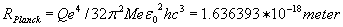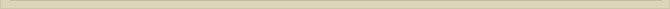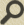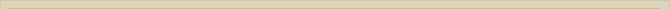## The photon and the constant of Planck mathematically

When you are interested in physics you must read “Unbelievable“!

A photon, with enough energy, can transform, split up into a positron and an electron. The assumption that a photon can be described as two charges in oscillation seems acceptable. The Coulomb-force between the two charges is: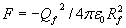If we try to calculate the energy of the oscillation with F we have to solve a few problems first. Unknown are the size of the charges Qf, the distance Rf at the beginning of the oscillation and at what distance F becomes inactive (so the singularity is avoided).

We assume the maximum distance between the two charges Rf is ¼ λ. The force is then:This is the total force between both charges. When +Qf moves R to –Qf, then –Qf also moves R to +Qf. The force while both particles moved R will be: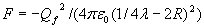When we integrate F to a distance R=0 a singularity arises. Both the force and energy become infinite. This problem was not solvable before. Now we have an indication for the distance to which F is active: the radius of the neutron Rn or the radius of the proton Rp.

The diameter, the distance between +Qf and –Qf when F=0, when both charges penetrate the point-volume, is twice the radius of the point-volume and therefore approx. twice the calculated radius (2Rn) of the neutron or proton. The energy of the oscillation   will be: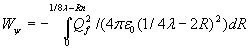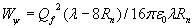The last formula demonstrates that the arbitrary distance Rf=1/4λ , the maximum distance between the two opposite charges,  is of no influence for the energy Wψ of the oscillation because 8Rn<<λ. The oscillation energy will be: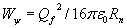The only unknown factor is Qf. We assume that Qf=Qe/n where n is an arbitrary number: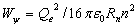The charge Qf is replaced  Qe/n. The unknown factor is now n. The most straightforward assumption is that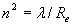, because the radius of the electron with charge –Qe is Re and the wavelength λ has to be proportional with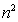. Substitution gives: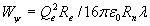Like electron, proton and neutron the photon has two degrees of freedom to store energy: oscillation and movement. So the total energy of the photon Wf will be equal to 2Wψ.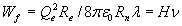while the energy of the photon is:

Wf  = hν

with H the derived constant and h the constant of Planck. The deviation between h and H is 6%, so the relation is not exact, but fair enough when one considers the radius of the neutron is playing a crucial part. The coincidence that the constant of Planck will derived within a 6% accuracy, when the ether is no more than a wild fantasy, is very unlikely. There are many possible causes to explain the difference, like:

• The mathematical constant π and the natural logarithm e have to arise somewhere. Maybe they arise in the point-volume?
• The electric field of the with speed c moving charges of the photon is forced in a two dimensional circle. Is the three dimensional equation for the electrostatic attraction still completely valid?
• Can the point-volume be transformed in some way when it becomes a neutron?

The picture the ether showed us so far is that the point-volume is not rigid.

We assume that the constant of Planck (h) determines the radius of the point-volume rather than the calculated, and probably deformed, radius of the proton or neutron.

When we use the above derived formulaand replace H with the experimental derived value for Planck’s constant h we can calculate Rplanck; the radius of the point-volume according to the experimental value of h: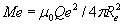and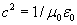, becomes: